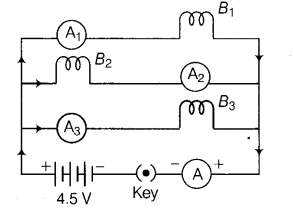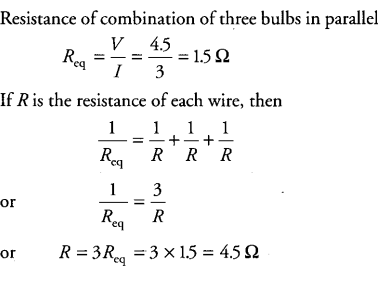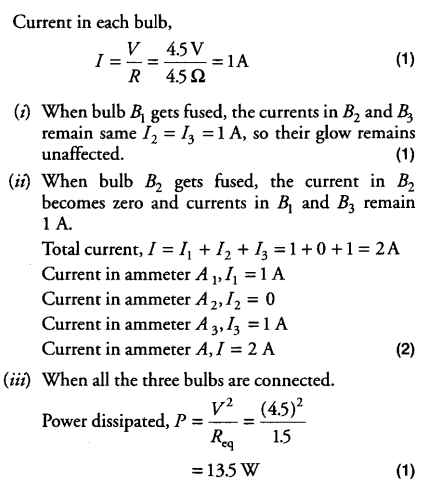# \$B_{1}\$, \$B_{2}\$ and \$B_{3}\$ are three identical bulbs connected as shown in figure

B_{1}, B_{2} and B_{3} are three identical bulbs connected as shown in figure. Ammeters A_{1}, A_{2} and A_{3} connected as shown. When all the bulbs glow, the current of 3 A is recorded by ammeter A.(i) What happens to the glow of the other two bulbs when bulb B_{1} gets fused?
(ii) What happens to the reading of A_{1}, A_{2} and A_{3} and A when the bulb B_{2} gets fused?
(iii) How much power is dissipated in the circuit when all the three bulbs glow together?